# Octahedral & Tetrahedral Complexes Notes | Study Inorganic Chemistry - Chemistry

## Chemistry: Octahedral & Tetrahedral Complexes Notes | Study Inorganic Chemistry - Chemistry

The document Octahedral & Tetrahedral Complexes Notes | Study Inorganic Chemistry - Chemistry is a part of the Chemistry Course Inorganic Chemistry.
All you need of Chemistry at this link: Chemistry

Octahedral Complexes

Inner Orbital Complexes:

[Co(CN)6]3-: In this complex the oxidation state (O.S.) of cobalt is +3. The valence shell electronic configuration is 3d6. The CN- ligands are strong, therefore pairing of 3d electrons takes place. This lead to the formation of d 2sp3 configuration with the combination of two 3d, 4s and three 4p orbitals. These six hybrid orbitals overlap with six filled orbitals of ligands forming six coordinate bonds. The geometry of the complex is octahedral and since there are no unpaired electrons left, this complex is diamagnetic.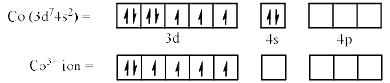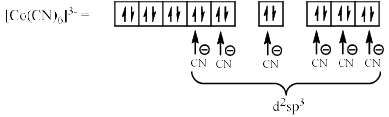Mn(CN)6]3–: In this complex the O.S. of Mn is +3 and its valence shell configuration is 3d4. Since CN is a strong field complex, pairing takes place according to the Hund’s rule of maximum multiplicity.
The pairing lead to the formation of six d2sp3 hybrid orbitals thereb y forming an octahedral complex. Since there are two unpaired electrons in the complex, it is paramagnetic.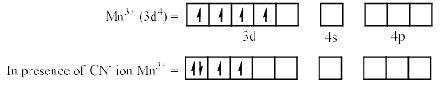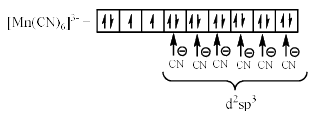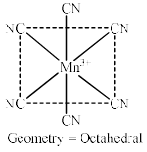The magnetic moment for the above complexes can be calculated by the following formula: Magnetic moment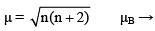Bohr’s magneton,         where  n = no. of unpaired e-s

For example, for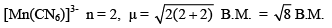= 2.82 B.M., (paramagnetic)

[V(NH3)6]3+: In this ion the O.S. of vanadium is +3 and its valence shell electronic configuration is 3d2. Alt hough, the NH3 is a strong ligand, this will not lead to the pairing of electrons because there are enough inner orbitals available for hybridisation. The orbitals combine to form six d2sp3 hybrid orbitals having octahedral geometry. The complex is paramagnetic since there are two unpaired electrons.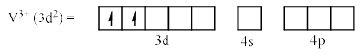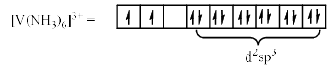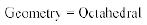n = 2,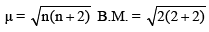= B.M. = 2.88 B.M., (paramagnetic)

Outer Orbital Complexes:

[CoF6]3–: In this the outer shell electronic configuration of cobolt is 3d6. F is a weak ligand, hence there will be no pairing of 3d electrons of the metal cation. This led to the formation of six sp3d2 orbitals with the help of outer 4d orbitals. The geometry is octahedral and the complex is paramagnetic with correspondence to four unpaired electrons.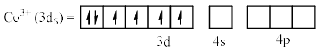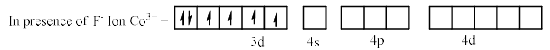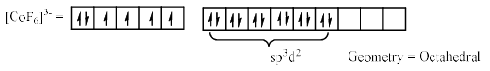n = 4,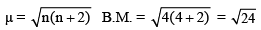= 4.90 B.M. (paramagnetic)

[Fe[H2O]5(NO)]2+ ion: This complex is formed in the blue ring test of nitrate ion. In this the o.s. of Fe is +1 because NO exist in +1 oxidation state in complexes of Fe. Hence the valence shell electronic configuration is 3d64s1. The H2O is a weak field ligand where as NO+ is a strong field ligand. This single NO+ ligand lead to the pairing of only two unpaired electrons, thereby making valence shell electronic configuration 3d6. Therefore sp3dhybridisation takes place and an octahedral complex is formed. The magnetic moment corresponds to the four unpaired electrons.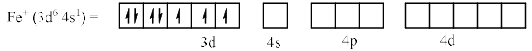In presence of Noligand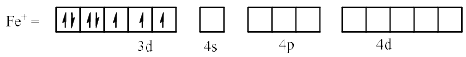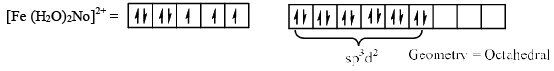n = 3,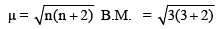B.M. = 3.87 B.M. (paramagnetic)

Tetrahedral Complexes

Tetrahedral complexes are either sp3 or sd3 hybridised. Examples are shown below.

[NiCl4]2–In this the outer shell electronic configuration of Ni (+2 o.s.) is 3d8. Since Cl is a weak ligand, pairing of 3d electrons does not take place. None of the 3d orbitals are vacant. The vacant 4s and 4p orbitals hybridised to give four equivalent sp3 hybrid orbitals thereb y forming tetrahedral geometry. There are two unpaired electrons. Hence, the magnetic moment of the complex is 2.44.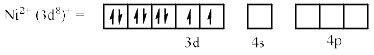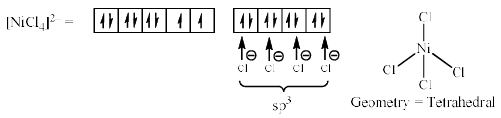n = 2,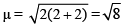= 2.82  B.M. (paramagnetic)

[Ni(CO)4]: In this complex the o.s. of Ni is zero. Hence the electronic configuration of outermost shell is 3d84s2. Since CO is a strong ligand, pairing of electrons do occur and after pairing there is no vacant 3d orbitals left. Thus the vacant orbital available for hybridisation are 4s and 4p which combine to give four sp3 hybrid orbitals. This will form tetrahedral geometry and the magnetic moment is zero as there are no unpaired electrons. Therefore the complex is diamagnetic.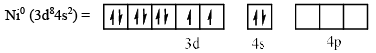In presence of CO ligand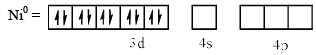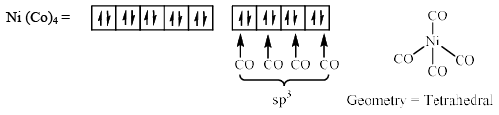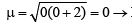Diamagnetic

KMnO4: In the MnO4– complex ion, the o.s. of Mn is +7. The ground state configuration of M n is 3d54s2. In Mn7+ ion all the five 3d, 4s-orbitals are vacant. The vacant 4s and 3d orbitals hybridised to give sd3 hybrid orbitals. Thus Mn7+ ion is sd3 hybridised and form tetrahed ral geometry in t his complex ion as shown below: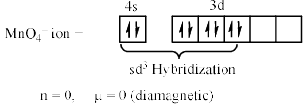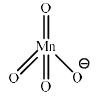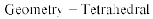Similar arrangement is also observed for K2Cr2O7 (Cr2O72- ion).

The document Octahedral & Tetrahedral Complexes Notes | Study Inorganic Chemistry - Chemistry is a part of the Chemistry Course Inorganic Chemistry.
All you need of Chemistry at this link: ChemistryUse Code STAYHOME200 and get INR 200 additional OFF

## Inorganic Chemistry

49 videos|71 docs|16 tests

Track your progress, build streaks, highlight & save important lessons and more!

,

,

,

,

,

,

,

,

,

,

,

,

,

,

,

,

,

,

,

,

,

;